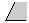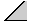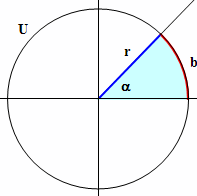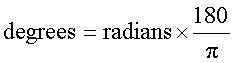Deutsche VersionType your value in a box, click your mouse anywhere on the page, or press tab key.
Power of 10 notation can be used as follows: 3.2×104 is entered 3.2e4

The natural unit for measuring angles is the radian, which is derived from the formula for
the circumference c of a circle: c = 2 π × r, where r is the radius. If r = 1, then c = 2 π,
so the radian is defined such that a circle has 2 π radians. It also has 360 degrees.
1 radian = 360 / 2 π = 180 / π =57.295779513082320876798154814106 degrees
.

 Angle Degrees Part of Pi in numbers90° π/2 1.57079632760° π/3 1.04719755145° π/4 0.78539816330° π/6 0.523598775Formulas